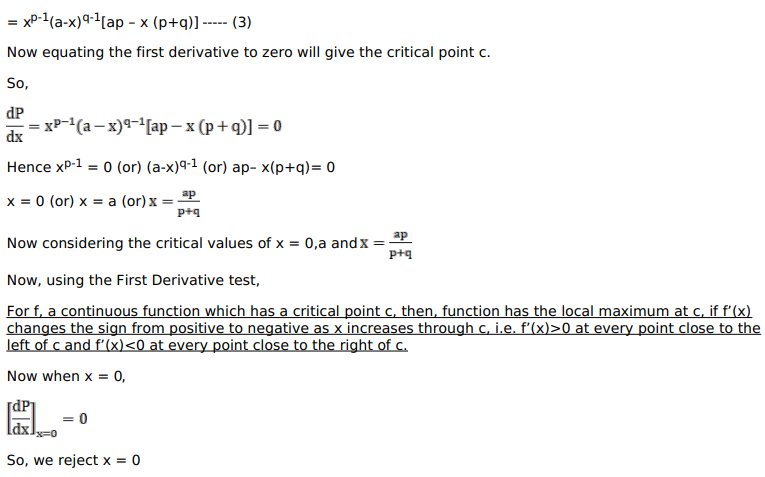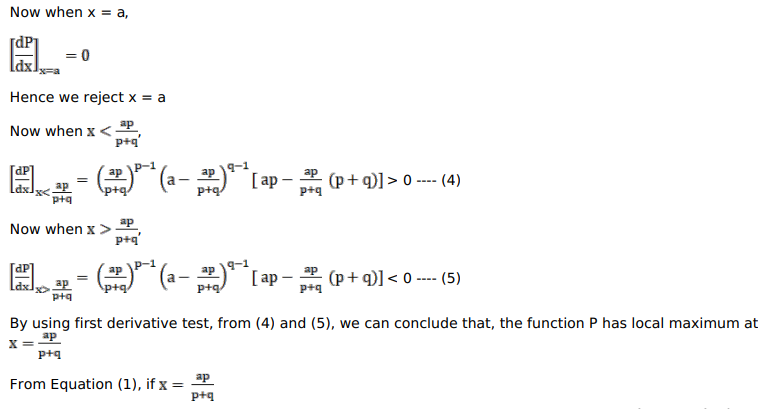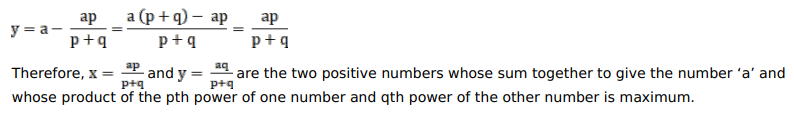# Divide a into two parts such that the product of

Question:

Divide a into two parts such that the product of the pth power of one part and the qth power of the second part may be maximum.

Solution: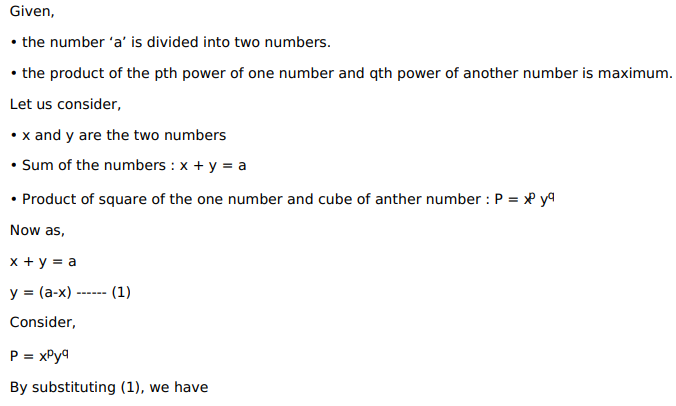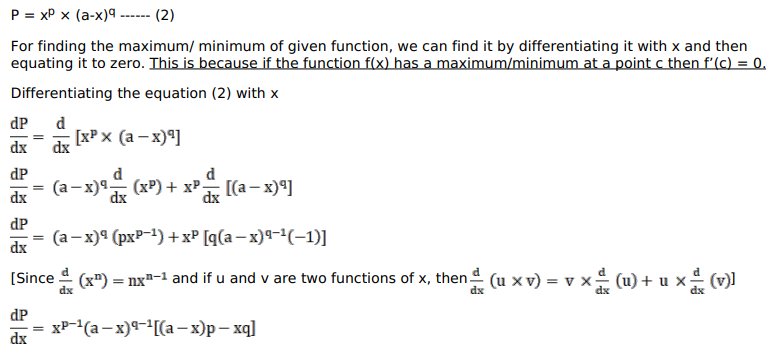$=x^{p-1}(a-x)^{q-1}[a p-x p-x q]$